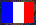# Floating-Point Arithmetic Test Programs

Here are a few C programs to test the floating-point arithmetic of your machine:

tst-ieee754.c

Miscellaneous tests of the floating-point arithmetic: test of the evaluation of the floating-point expressions in C (showing a bug in gcc when the processor is configured in extended precision, e.g. under Linux/x86), test of the addition and the multiplication with signed zeros, test of the power function `pow`. The results on various machines (compressed archive) and a partial summary (text file).

nearestint.c

Test of the nearest integer functions, in the four IEEE-754 rounding modes: cast to int type, `trunc`, `floor`, `ceil`, `round`, `nearbyint`, `rint`. These tests show a bug in the PowerPC implementation of the `rint` function in the glibc (fixed in the CVS on 6 January 2005).

testatan.c

Test of the arc tangent function `atan` (see Debian bug 210613).

testlog.c

Test of the logarithm function `log` (see Debian bug 210400).

testsin.c

Test of the sine function `sin`.

overflow.c

Test of the overflow behavior with gcc and intermediate extended precision (e.g. under Linux/x86).

testlgamma.c

Test of the gamma functions `tgamma`, `lgamma` and `lgamma_r`.

remquo.c

Test of the `remquo` function.

fma-tests.c

Test of the `fma` function (see glibc bug 3268 and Debian bug 372544).

contract2fma.c

Test the effect of the contraction to FMA by computing `sqrt (a * a - b * b)` with a = b (the code is written in such a way that this equality should not be used for optimizing at compile time). Under some conditions, the ISO C99 standard allows floating expressions to be contracted: for instance, the expression `x * y + z` can be contracted into a single operation (with only one rounding) on processors that have a FMA. In general, this improves the accuracy of the numerical computations, but this can also have the opposite effect, and even yield side effects, as in the above code, where the symmetry is broken by the introduction of a FMA. That's why the contraction of floating expressions can be disabled by the FP_CONTRACT pragma; in this case, the result of this program should always be 0. But GCC up to 4.8 ignores FP_CONTRACT pragma set to OFF, so that the program gives incorrect results on PowerPC, IA-64 (Itanium), and recent x86_64 processors. Worse, even on the general (non-trivial and possibly useful in practice) case `a >= b ? sqrt (a * a - b * b) : 0`, one can get a NaN.

For instance, when running contract2fma (compiled with GCC 4.8 or below on one of the architectures mentioned above) on 1, 1.1 and 1.2, one gets:

```Test of a >= b ? sqrt (a * a - b * b) : 0 with FP_CONTRACT OFF
test(1) = 0
test(1.1000000000000000888) = 2.9802322387695326562e-09
test(1.1999999999999999556) = nan```

Note: With GCC 4.9 and later, one needs to compile in ISO C mode (-std=c99 or -std=c11) to have contraction of floating expressions disabled by default (necessary since GCC still ignores the FP_CONTRACT pragma).

rndmode.c

Measure the `fegetround`/`fesetround` performance when the rounding mode doesn't change. See glibc very old thread, and in particular this message.

transf-generated.c

Test various simple expressions that can lead to transformations by the compiler in order to optimize. Such transformations are valid on the real numbers, but can here be invalid due to special IEEE-754 values (NaN, infinities, signed zeros).

The results can be affected by compiler options related to optimization of floating-point expressions. See an example of use with GCC (details about floating-point with GCC). In errors, y0 is the obtained value and y1 is the expected (correct) value. Note: it is assumed that the `volatile` qualifier has the effect to disable the optimizations, otherwise nothing is tested (see the source).

This C file is actually generated from transformations.c with the gen-transf Perl script.

webmaster@vinc17.org